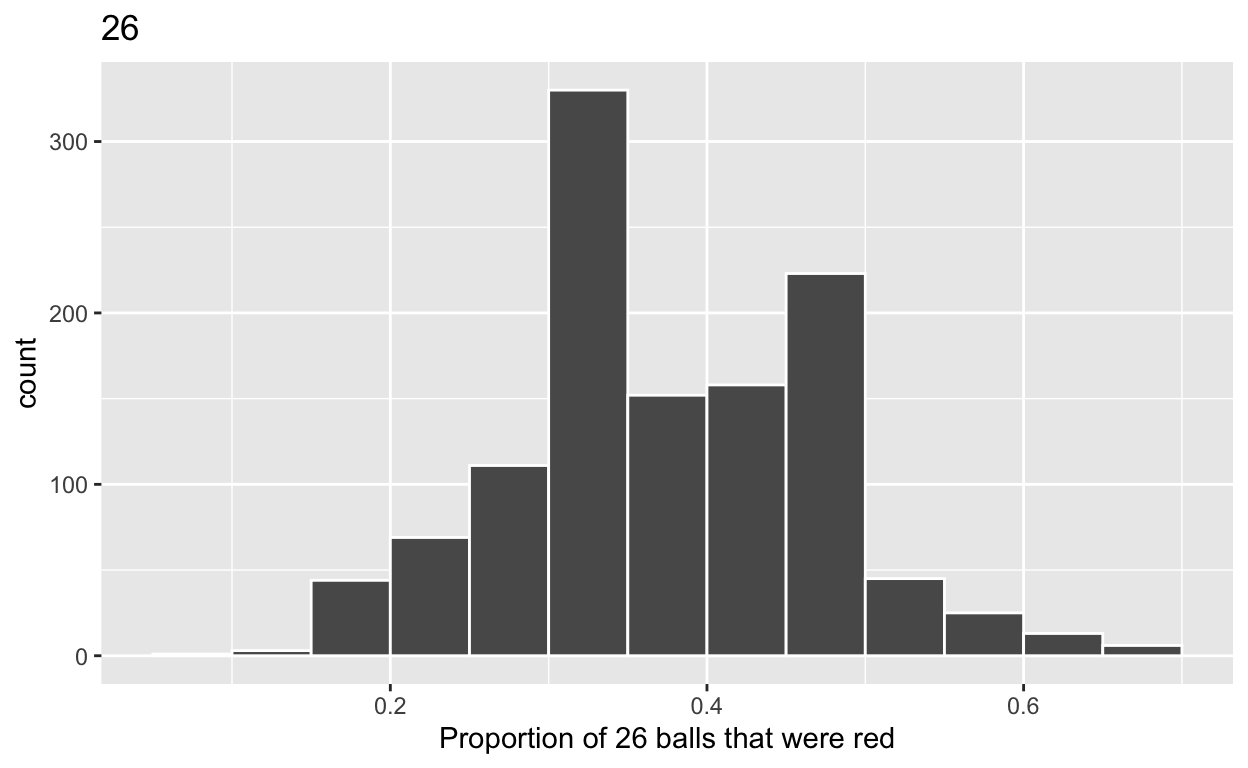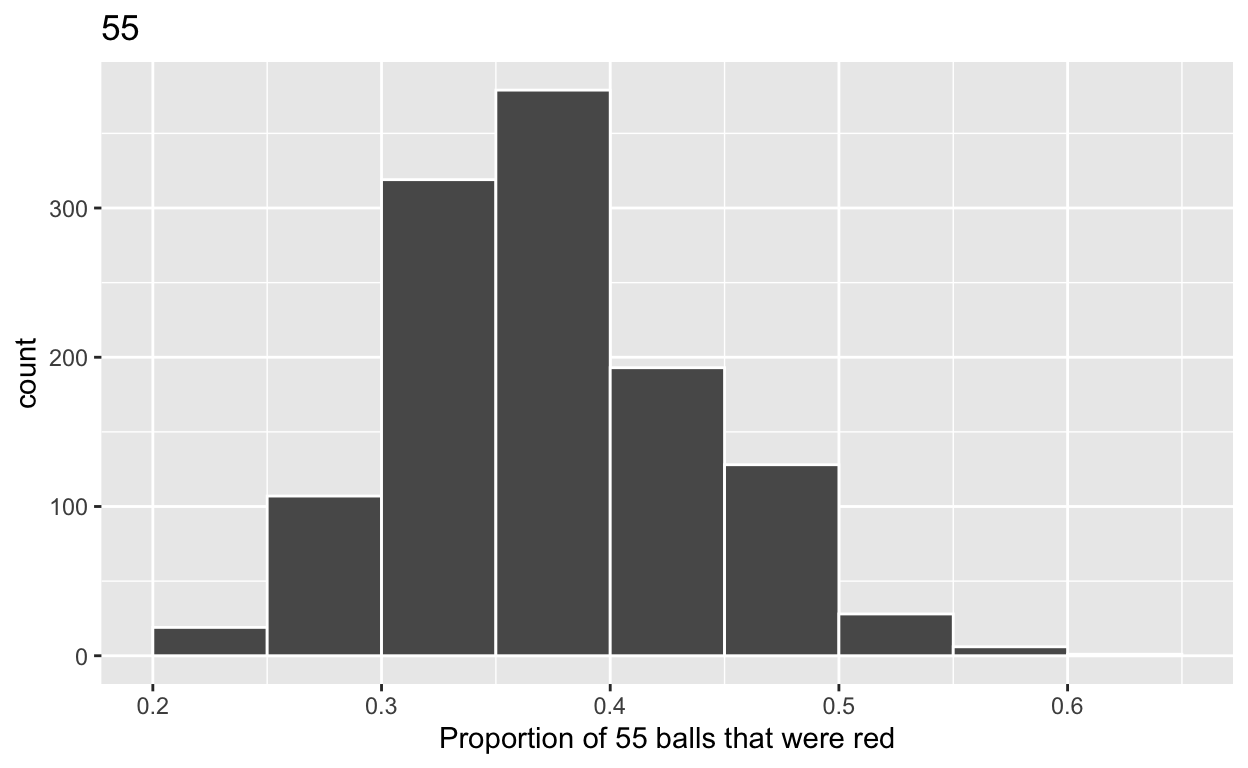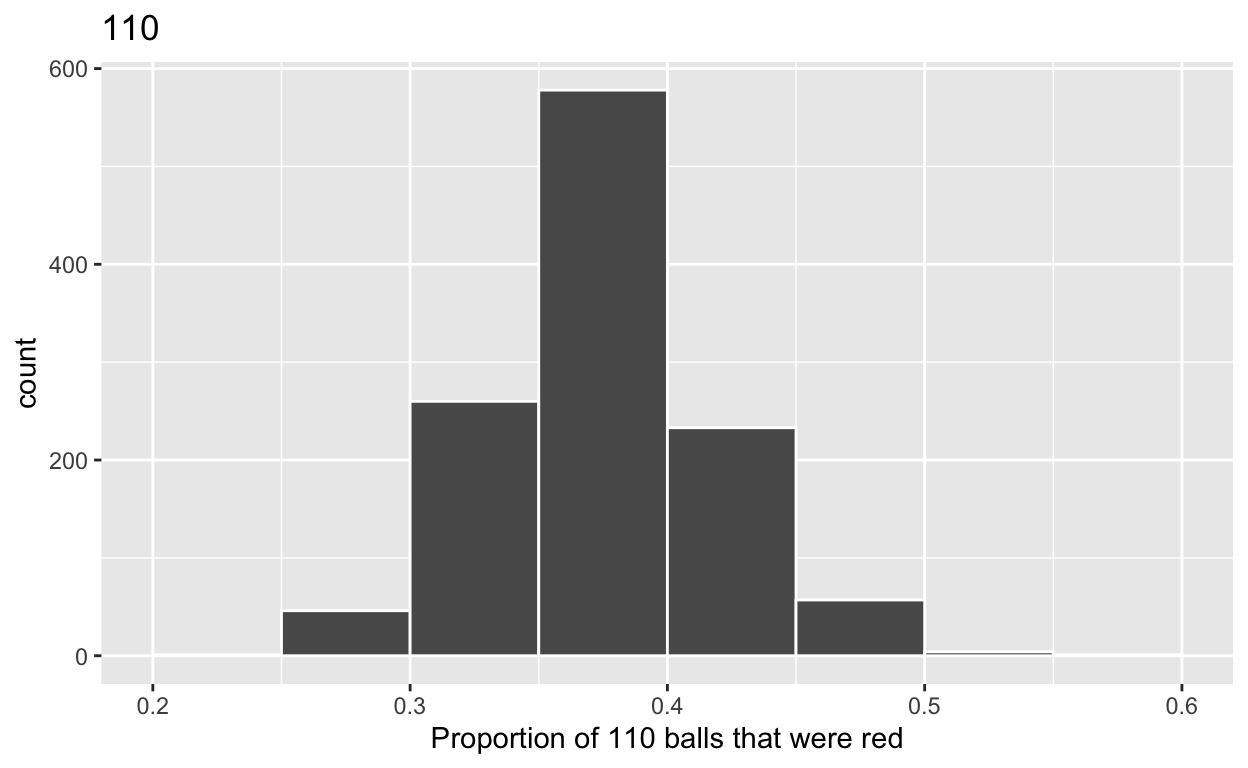# Sampling

A short description of the post.

1. Load the R package we will use.
``````library(tidyverse)
library(moderndive)
``````
1. Quiz questions
• Replace all the instances of ‘SEE QUIZ’. These are inputs from your moodle quiz.

• Replace all the instances of ‘???’. These are answers on your moodle quiz.

• Run all the individual code chunks to make sure the answers in this file correspond with your quiz answers

• After you check all your code chunks run then you can knit it. It won’t knit until the ??? are replaced

• The quiz assumes that you have watched the videos and worked through the examples in Chapter 7 of ModernDive

# Question:

7.2.4 in Modern Dive with different sample sizes and repetitions

• Make sure you have installed and loaded the tidyverse and the moderndive packages

• Fill in the blanks

• Put the command you use in the Rchunks in your Rmd file for this quiz.

Modify the code for comparing differnet sample sizes from the virtual bowl

Segment 1: sample size = 26

1.a) Take 1180 samples of size of 26 instead of 1000 replicates of size 25 from the bowl dataset. Assign the output to virtual_samples_SEE QUIZ
``````virtual_samples_26  <- bowl  %>%
rep_sample_n(size = 26, reps = 1180)
``````

1.b) Compute resulting SEE QUIZ replicates of proportion red

• group_by replicate THEN
• create variable red equal to the sum of all the red balls
• create variable prop_red equal to variable red / SEE QUIZ
• Assign the output to virtual_prop_red_SEE QUIZ
``````virtual_prop_red_26 <- virtual_samples_26 %>%
group_by(replicate) %>%
summarize(red = sum(color == "red")) %>%
mutate(prop_red = red / 26)
``````

1.c) Plot distribution of virtual_prop_red_SEE QUIZ via a histogram

• use labs to

• label x axis = “Proportion of SEE QUIZ balls that were red”

• create title = “SEE QUIZ”

``````ggplot(virtual_prop_red_26, aes(x = prop_red)) +
geom_histogram(binwidth = 0.05, boundary = 0.4, color = "white") +
labs(x = "Proportion of 26 balls that were red", title = "26")
``````2.a) Take SEE QUIZ samples of size of SEE QUIZ instead of 1000 replicates of size 50. Assign the output to virtual_samples_SEE QUIZ

Segment 2: sample size = 55
``````virtual_samples_55  <- bowl  %>%
rep_sample_n(size = 55, reps = 1180)
``````

2.b) Compute resulting SEE QUIZ replicates of proportion red

start with virtual_samples_SEE QUIZ THEN group_by replicate THEN create variable red equal to the sum of all the red balls create variable prop_red equal to variable red / SEE QUIZ Assign the output to virtual_prop_red_SEE QUIZ

``````virtual_prop_red_55 <- virtual_samples_55 %>%
group_by(replicate) %>%
summarize(red = sum(color == "red")) %>%
mutate(prop_red = red / 55)
``````

2.c) Plot distribution of virtual_prop_red_SEE QUIZ via a histogram

use labs to

label x axis = “Proportion of SEE QUIZ balls that were red” create title = “SEE QUIZ”

``````ggplot(virtual_prop_red_55, aes(x = prop_red)) +
geom_histogram(binwidth = 0.05, boundary = 0.4, color = "white") +
labs(x = "Proportion of 55 balls that were red", title = "55")
``````Segment 3: sample size = SEE QUIZ

3.a) Take SEE QUIZ samples of size of SEE QUIZ instead of 1000 replicates of size 50. Assign the output to virtual_samples_SEE QUIZ

``````virtual_samples_110  <- bowl  %>%
rep_sample_n(size = 110, reps = 1180)
``````

3.b) Compute resulting SEE QUIZ replicates of proportion red

start with virtual_samples_SEE QUIZ THEN group_by replicate THEN create variable red equal to the sum of all the red balls create variable prop_red equal to variable red / SEE QUIZ Assign the output to virtual_prop_red_SEE QUIZ

``````virtual_prop_red_110 <- virtual_samples_110 %>%
group_by(replicate) %>%
summarize(red = sum(color == "red")) %>%
mutate(prop_red = red / 110)
``````

3.c) Plot distribution of virtual_prop_red_SEE QUIZ via a histogram

use labs to

label x axis = “Proportion of SEE QUIZ balls that were red” create title = “SEE QUIZ”

``````ggplot(virtual_prop_red_110, aes(x = prop_red)) +
geom_histogram(binwidth = 0.05, boundary = 0.4, color = "white") +
labs(x = "Proportion of 110 balls that were red", title = "110")
``````Calculate the standard deviations for your three sets of SEE QUIZ values of prop_red using the standard deviation

n = 26

``````virtual_prop_red_26  %>%
summarize(sd = sd(prop_red))
``````
``````# A tibble: 1 x 1
sd
<dbl>
1 0.0995``````

n = 55

``````virtual_prop_red_55  %>%
summarize(sd = sd(prop_red))
``````
``````# A tibble: 1 x 1
sd
<dbl>
1 0.0639``````

n = 110

``````virtual_prop_red_110  %>%
summarize(sd = sd(prop_red))
``````
``````# A tibble: 1 x 1
sd
<dbl>
1 0.0435``````